# Deep Learning 101 —  Neural Networks Explained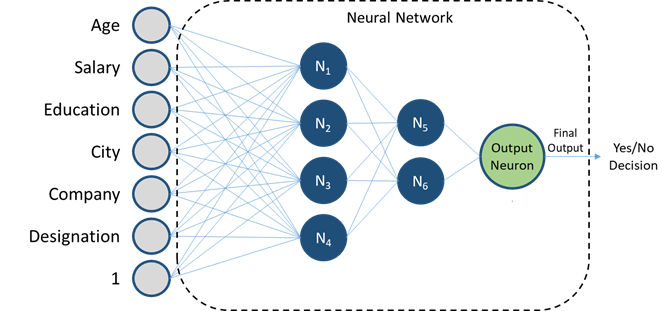The past few decades have witnessed a massive boom in the penetration as well as the power of computation, and amidst this information.

The past few decades have witnessed a massive boom in the penetration as well as the power of computation, and amidst this information revolution, AI has radically changed the way people and businesses make decisions. The poster-boys for these cutting edge AI solutions — Deep Learning and Neural Networks, despite their hype, are understood only by a few.

In this article, I will try to explain what Neural Networks are, and how they function, and their strengths and limitations. And hopefully, convince you that they’re much simpler than they’re thought to be.

_NOTE: Deep Learning is an subset of Machine Learning, and if you’re unsure how that works, I’d advise you check out [this article_]

Before diving right in, let’s explore a simpler problem, to establish a few fundamental concepts.

# Logistic Regression

Assume we’re a credit card company targeting people without an adequate credit history. Using the historical data of its users, we want to decide whether a new applicant should be given the credit card or not.

Assume the data we have to make the decision is the following.

· Age

· Salary

· Education

· City

· Company

· Designation

Note: I realize that the latter 4 are “qualitative” variables, but for this illustration, let’s assume we can somehow quantify them

The final decision is a Yes or No, thus making it a classification problem. To solve this we’ll make a small modification to our linear regression approach — After multiplying the variables with their weights (the technically correct name of the slopes or coefficients we used in Linear Regression) and adding them, (and the constant), the result can be any number, and to convert it into a Yes/No decision, we will have to process it further, i.e. pass it through a function.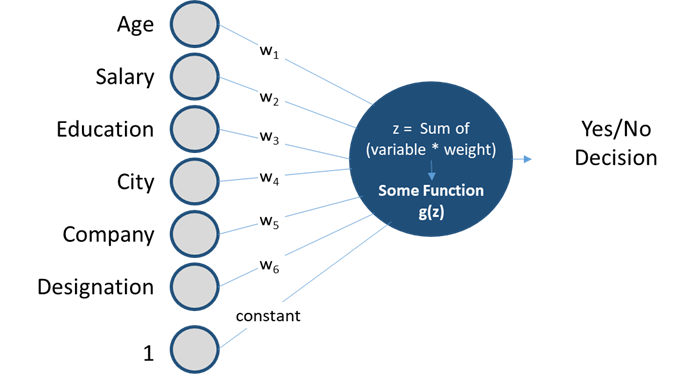One option for g could be a simple Threshold function:

![Threshold Function]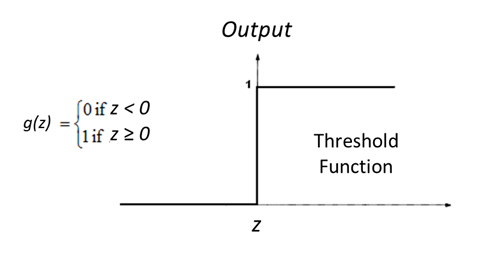Ie. The decision being No if the sum product of variables and weights is z<0 and Yes if Z>=0

However often, instead of absolute Yes or No, we may want the probability of the result, and also because the algorithm computing the optimum weights work better for smooth (or in technical terms, differentiable) functions, we use a different function called the sigmoid.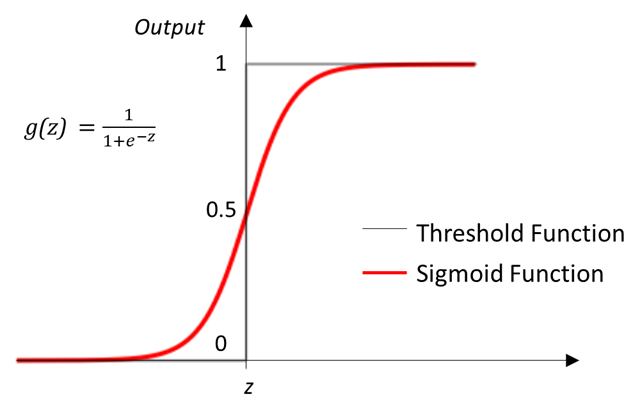Tying it all together, for a new customer, we can input the different variables (age, salary etc.), sum their product with them with the weights, and apply the sigmoid function to get the probability of his eligibility for the card, and this whole process is called Logistic Regression.

# Activation Functions

The reason for taking the detour through Logistic Regression was to introduce the concept of activation functions. The threshold or sigmoid function used in Logistic Regression were examples of activation functions.

Any function applied on the sum-product of variables and weights(note that there will be a corresponding weight for each variable) is known as an activation function. Apart from the Threshold and sigmoid functions discussed above, a few others that are commonly used

## ReLU (Rectified Linear Unit):

![ReLU graph]

This means returning Z as it is if it’s positive, and returning 0 otherwise.

## Tanh (Hyperbolic Tan):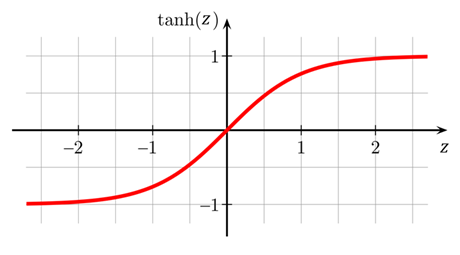This is sigmoid stretched downwards on the y-axis so that it’s centred at (0,0) instead of (0,0.5)

These, along with their small variations are the most commonly used activation functions in Neural Networks because of reasons beyond the scope of this article.

## Most popular Data Science and Machine Learning courses — July 2020

Most popular Data Science and Machine Learning courses — August 2020. This list was last updated in August 2020 — and will be updated regularly so as to keep it relevant

## Cheat Sheets for AI, Neural Networks, Machine Learning, Deep Learning & Big Data

Cheat Sheets for AI, Neural Networks, Machine Learning, Deep Learning & Big Data

## Artificial Neural Networks — Recurrent Neural Networks

Artificial Neural Networks — Recurrent Neural Networks. Remembering the history and predicting the future with neural networks. A intuition behind Recurrent neural networks.

## Fundamentals of Neural Network in Machine Learning

Fundamentals of Neural Network in Machine Learning. What is a Neuron? What is the Activation Function? How do Neural Network Works? How do Neural Networks Learn?

## Artificial Intelligence vs. Machine Learning vs. Deep Learning

Simple explanations of Artificial Intelligence, Machine Learning, and Deep Learning and how they’re all different